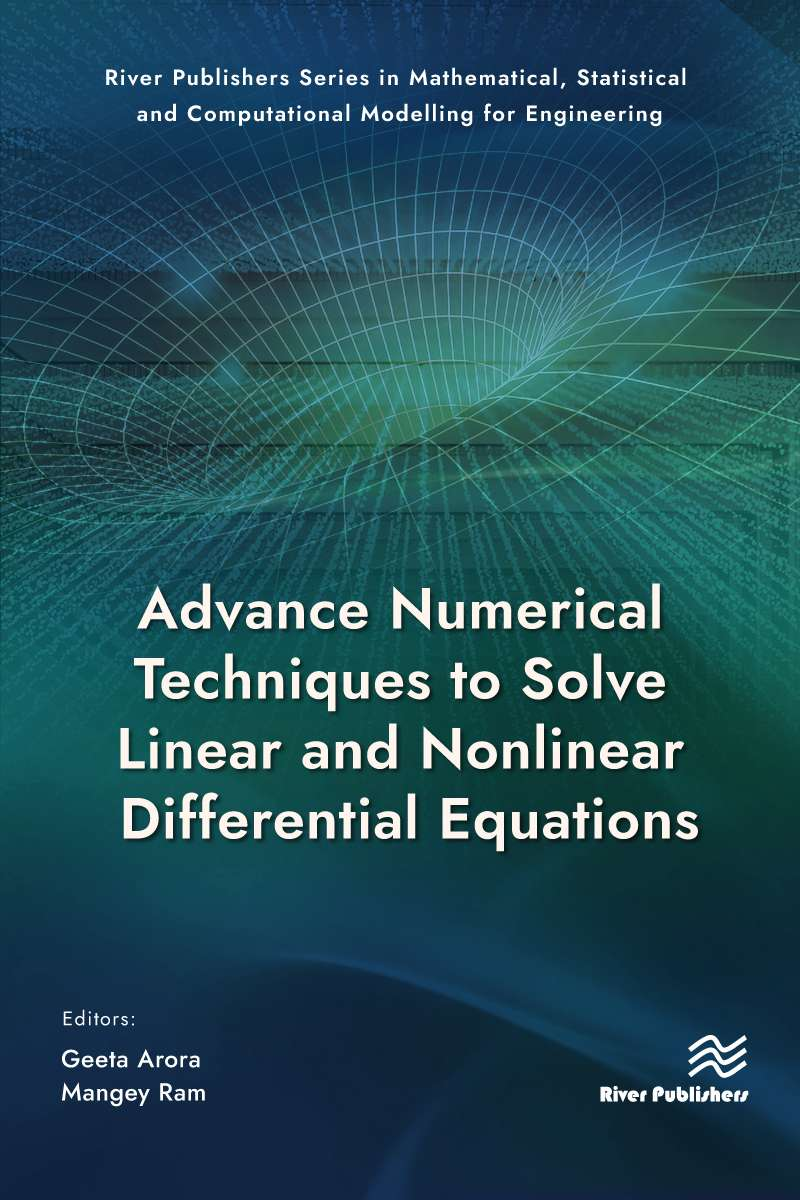Advance Numerical Techniques to Solve Linear and Nonlinear DifferentialEquations

River Publishers Series in Mathematical, Statistical and Computational Modelling for Engineering

##### Advance Numerical Techniques to Solve Linear and Nonlinear DifferentialEquations Forthcoming

Editors:
Geeta Arora,Lovely Professional University, Punjab, India
Mangey Ram, Graphic Era (Deemed to be University), Dehradun, India

ISBN: 9788770229876 e-ISBN: 9788770229869

Available: October 2023

Real-world issues can be translated into the language and concepts of mathematics with the use of mathematical models. This book providesthese real-world examples, explores research challenges in numerical treatment, and demonstrates how to create new numerical methods for resolving problems.Theories and practical applications in the sciences and engineering are also discussed.Students of engineering and applied mathematics, as well as researchers and engineers who use computers to solve problems numerically or oversee those who do, will find this book focusing on advance numerical techniques to solve linear and nonlinear differential equations useful.
Models guided by differential equations with intuitive solutions can be used throughout engineering and the sciences. Almost any changing system may be described by a set of differential equations. They may be found just about anywhere you look in fields including physics, engineering, economics, sociology, biology, business, healthcare, etc. The nature of these equations has been investigated by several mathematicians over the course of hundreds of years and, consequently, numerous effective methods for solving them have been created. It is often impractical to find a purely analytical solution to a system described by a differential equation because either the system itself is too complex or the system being described is too vast. Numerical approaches and computer simulations are especially helpful in such systems.
Integrability Conditions; Differential Quadrature Method;Fuzzy Linear Fractional Programming Problem; Orthogonal Collocation on Finite Elements; B-spline Basis Function; CFDS for KleinâGordon Equation; Sumudu ADM;Crystal Lattice Theory;Residual Power Series Method.